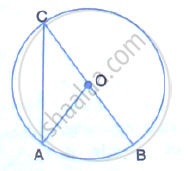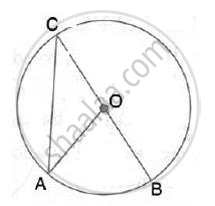Share

Given O is the Centre of the Circle and ∠Aob = 70°. Calculate the Value Of: (I) ∠Oca . (Ii) ∠Oca . - ICSE Class 10 - Mathematics

ConceptChord Properties - Chords Equidistant from the Center Are Equal (Without Proof)

Question

Given O is the centre of the circle and ∠AOB  = 70°. Calculate the value of:
(i)  ∠OCA  .
(ii) ∠OCA  .SolutionHere, ∠AOB= 2∠ACB
(Angle at the center is double the angle at the circumference by the same chord)
⇒  ∠ACB =70/2 =35°
Now, OC = OA (radii of same circle)
⇒ ∠OCA = ∠OAC =35°

Is there an error in this question or solution?

Video TutorialsVIEW ALL 

Solution Given O is the Centre of the Circle and ∠Aob = 70°. Calculate the Value Of: (I) ∠Oca . (Ii) ∠Oca . Concept: Chord Properties - Chords Equidistant from the Center Are Equal (Without Proof).
S